Education

# Cash Flow Study For Maui Rental Property

In an article dated June 19, 2019, The Wall Street Journal reported that according to data released by CoreLogic, Inc., the share of homes purchased by investors in the United States reached 11.3%, the highest on record.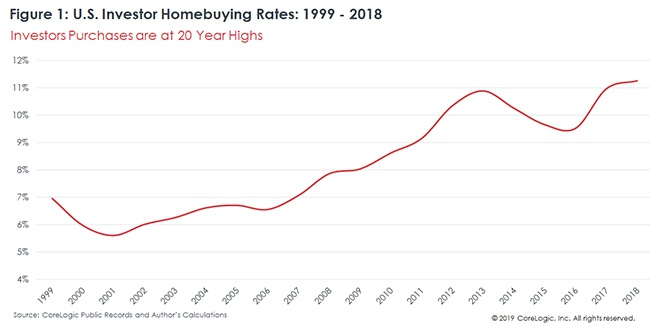Here on Maui, the share of homes purchased by investors is closer to 30-35% according to local Realtor opinion. Personally, from my last 32 sales, 12 were Investor Purchases, making up 37.5%

If you are looking to invest in Maui real estate as investment rental property, it is important to understand cash flow and pertinent planning strategies.

The Following Example Will Cover These Important Topics Relevant to Investor Purchases:

Measuring Housing Inventory

• In Terms of Months of Inventory

Measuring Profit

• Income/Cash Flow Method
• Solving for Capitalization Rate (CAP Rate)
• Solving for Property Value
• Solving for Monthly Rent
• Solving for Net Operating Income (NOI)
• Appreciation in Value Method
• Internal Rate of Return (IRR)

Leverage

• In Terms of Mortgage Rate

### Housing Inventory

(In Terms of Months of Inventory)

To determine housing inventory in terms of “Months of Inventory”, take the total number of homes listed for sale during the last month and divide that number by the total number of homes sold in that month. This determines the number of months of inventory remaining.

Housing Inventory Example

If there were 400 homes listed for sale in June, and 100 of these listings sold in June, then 400 divided by 100 equals 4 months of inventory.

• 6 months of inventory equals a Balanced Market.
• More than 6 months of inventory equals a Buyer’s Market.

Less than 6 months of inventory equals a Seller’s Market.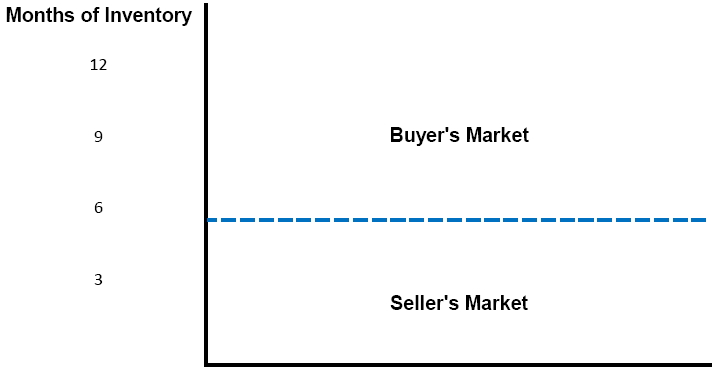### Measuring Profit

Two methods to profit with real estate:

• Income / Cash Flow
• Appreciation in Value

### Income / Cash Flow

Let’s assume that you are considering the purchase of a home on Maui and it is your intention to rent this unit on a Long-Term Basis (6 month minimum lease term).

Applicable Terms:

• Gross Annual Rental Income
• Annual Expenses
• Net Operating Income (NOI)
• Annual Mortgage Payments
• Capitalization Rate (CAP Rate)
• Property Value (Purchase Price)

Gross Annual Rental Income minus Annual Expenses equals Net Operating Income (NOI)

\$40,200 = Gross Annual Rental Income
– \$7,262.64 = Annual Expenses
\$32,937.36 = Net Operating Income (NOI)

Net Operating Income (NOI) minus Annual Mortgage Payments equals Positive Cash Flow

\$32,937.36 = NOI
-\$24,911.88 = Annual Mortgage Payments
\$8,025.48 = Annual Positive Cash Flow

Solving for Capitalization (CAP) Rate

Assume a List Price of \$567,500.

Net Operating Income (NOI) divided by Property Value (Purchase Price) = CAP Rate

NOI divided by Purchase Price = CAP Rate
\$32,937.36 ÷ \$567,500 = 5.804 % (Cap Rate)

Solving for Property Value

One of your goals with this potential purchase is a 6% CAP Rate; normal for this neighborhood.

NOI divided by CAP Rate = Purchase Price (In Order to Achieve CAP Rate Goal)
\$32,937.36 (NOI) ÷ 6% (Target CAP Rate) = \$548,956 (Purchase Price to Achieve 6% Cap Rate)

Solving for Monthly Rent

One of your goals is to determine the amount of rent necessary to achieve \$32,937.36/ month NOI.

Annual NOI Divided by 12 = Monthly NOI
\$32,937.36 ÷ 12 = \$2,744.78 (Monthly NOI)

Annual Expenses Divided by 12 = Monthly Expenses
\$7,262.64 ÷ 12 = \$605.22 Monthly Expenses

Monthly NOI plus Monthly Expenses = Gross Monthly Rent
\$2,744.78 + \$605.22 = \$3,350 (Gross Monthly Rent for \$32,937.36 Annual NOI and 6% CAP Rate)

Solving for NOI

Method to determine NOI.

Property Value X 6% CAP Rate = NOI
\$548,956 X 6% CAP Rate = \$32,937.36

A spreadsheet can be helpful computing the feasibility of a particular property. Here is an example: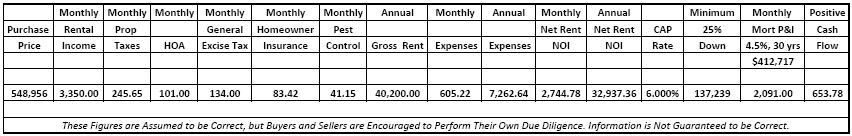### Appreciation In Value

Internal Rate of Return (IRR)

Using an Internal Rate of Return Calculator, The Rate of Return over time may be calculated.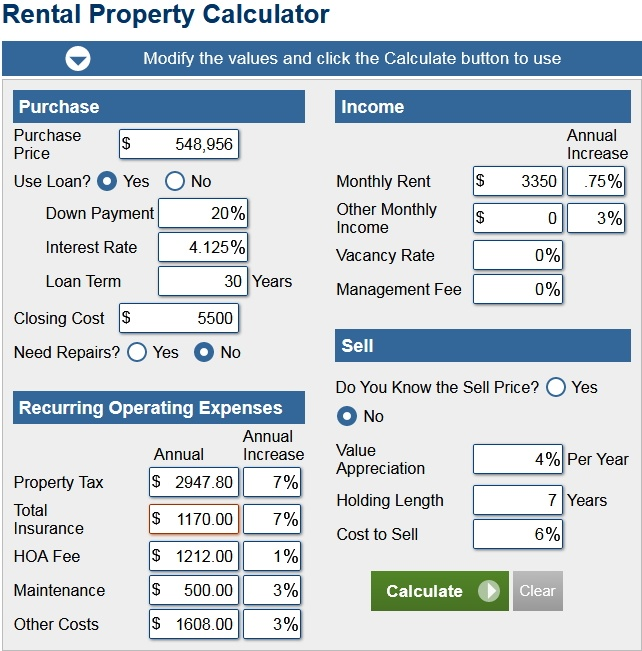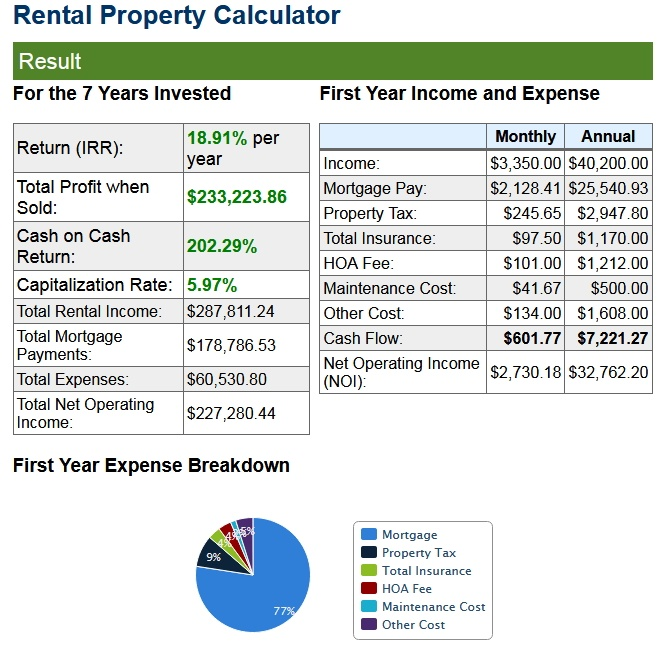Internal Rate of Return

Breakdown over Time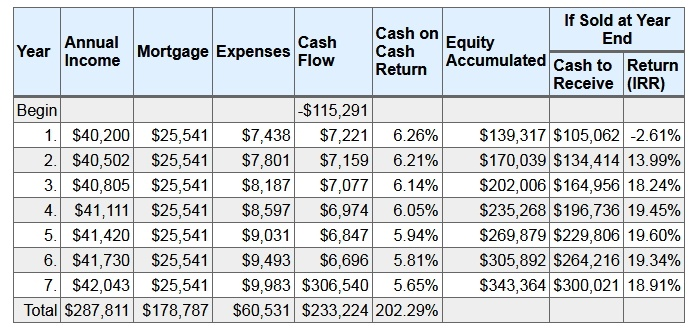### Leverage

Leveraging is a powerful tool.  Here is an example of leverage in terms of purchasing.

In this example, we have a purchase price of \$548,956. There is a down-payment of \$137,239.  We’ve borrowed \$412,717 in the form of a mortgage at 4.5% over 30 years. So, in essence, we have the opportunity to leverage a significant Internal Rate of Return with 75% of the purchase being with someone else’s money!

Yearly Rate of Return (if sold at year end)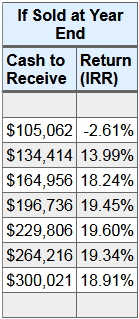But Wait! There’s More!

There Is Also The Potential To Leverage The Cost Of The Mortgage Interest!

In our example we are looking at a Rate of Appreciation of 4%, a CAP Rate of 6%, and Mortgage Interest Rate of 4%.

• CAP Rate Plus Appreciation Rate Lower Than Mortgage Rate = Negative Leverage
• CAP Rate Plus Appreciation Rate Higher Than Mortgage Rate = Positive Leverage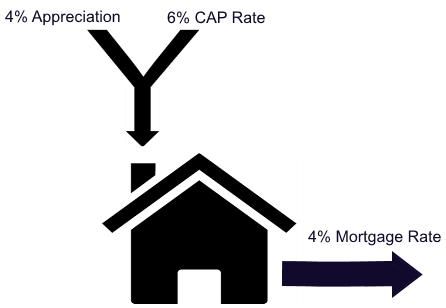6% CAP Rate Plus 4% Appreciation Rate = 10% Total

10% Total Minus 4% Mortgage Rate = 6% Positive Leverage

In conclusion, always remember to consult with your CPA and financial advisor!

These figures are assumed to be correct, but Buyers and Sellers are encouraged to Perform their Own Due Diligence. Information is Not Guaranteed to be Correct.